Search by Topic

Resources tagged with Maths Supporting SET similar to Random Squares:

Filter by: Content type:
Age range:
Challenge level:

There are 93 results

Broad Topics > Applications > Maths Supporting SETStats Statements

Age 16 to 18 Challenge Level:

Are these statistical statements sometimes, always or never true? Or it is impossible to say?Big and Small Numbers in the Living World

Age 11 to 16 Challenge Level:

Work with numbers big and small to estimate and calculate various quantities in biological contexts.Bessel's Equation

Age 16 to 18 Challenge Level:

Get further into power series using the fascinating Bessel's equation.Taking Trigonometry Series-ly

Age 16 to 18 Challenge Level:

Look at the advanced way of viewing sin and cos through their power series.Scale Invariance

Age 16 to 18 Challenge Level:

By exploring the concept of scale invariance, find the probability that a random piece of real data begins with a 1.Time to Evolve 2

Age 16 to 18 Challenge Level:

How is the length of time between the birth of an animal and the birth of its great great ... great grandparent distributed?Constantly Changing

Age 14 to 16 Challenge Level:

Many physical constants are only known to a certain accuracy. Explore the numerical error bounds in the mass of water and its constituents.Stirling Work

Age 16 to 18 Challenge Level:

See how enormously large quantities can cancel out to give a good approximation to the factorial function.Global Warming

Age 14 to 16 Challenge Level:

How much energy has gone into warming the planet?Building Approximations for Sin(x)

Age 16 to 18 Challenge Level:

Build up the concept of the Taylor series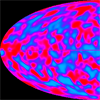Big and Small Numbers in Physics

Age 14 to 16 Challenge Level:

Work out the numerical values for these physical quantities.Big and Small Numbers in the Physical World

Age 14 to 16 Challenge Level:

Work with numbers big and small to estimate and calculate various quantities in physical contexts.Population Dynamics Collection

Age 16 to 18 Challenge Level:

This is our collection of tasks on the mathematical theme of 'Population Dynamics' for advanced students and those interested in mathematical modelling.Big and Small Numbers in Chemistry

Age 14 to 16 Challenge Level:

Get some practice using big and small numbers in chemistry.Approximately Certain

Age 14 to 18 Challenge Level:

Estimate these curious quantities sufficiently accurately that you can rank them in order of sizeDesigning Table Mats

Age 11 to 16 Challenge Level:

Formulate and investigate a simple mathematical model for the design of a table mat.The Wrong Stats

Age 16 to 18 Challenge Level:

Why MUST these statistical statements probably be at least a little bit wrong?Aim High

Age 16 to 18 Challenge Level:

How do you choose your planting levels to minimise the total loss at harvest time?Guessing the Graph

Age 14 to 16 Challenge Level:

Can you suggest a curve to fit some experimental data? Can you work out where the data might have come from?Integration Matcher

Age 16 to 18 Challenge Level:

Can you match the charts of these functions to the charts of their integrals?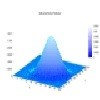Pdf Matcher

Age 16 to 18 Challenge Level:

Which pdfs match the curves?Whose Line Graph Is it Anyway?

Age 16 to 18 Challenge Level:

Which line graph, equations and physical processes go together?Vector Walk

Age 14 to 18 Challenge Level:

Starting with two basic vector steps, which destinations can you reach on a vector walk?Fix Me or Crush Me

Age 16 to 18 Challenge Level:

Can you make matrices which will fix one lucky vector and crush another to zero?Perfect Eclipse

Age 14 to 16 Challenge Level:

Use trigonometry to determine whether solar eclipses on earth can be perfect.Transformations for 10

Age 16 to 18 Challenge Level:

Explore the properties of matrix transformations with these 10 stimulating questions.Square Pair

Age 16 to 18 Challenge Level:

Explore the shape of a square after it is transformed by the action of a matrix.Matrix Meaning

Age 16 to 18 Challenge Level:

Explore the meaning behind the algebra and geometry of matrices with these 10 individual problems.Nine Eigen

Age 16 to 18 Challenge Level:

Explore how matrices can fix vectors and vector directions.Pdf Stories

Age 16 to 18 Challenge Level:

Invent scenarios which would give rise to these probability density functions.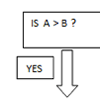Mystery Procedure

Age 14 to 16 Challenge Level:

Can you work out what this procedure is doing?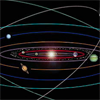Construct the Solar System

Age 14 to 18 Challenge Level:

Make an accurate diagram of the solar system and explore the concept of a grand conjunction.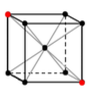Crystal Symmetry

Age 16 to 18 Challenge Level:

Use vectors and matrices to explore the symmetries of crystals.Dangerous Driver?

Age 16 to 18 Challenge Level:

Was it possible that this dangerous driving penalty was issued in error?A Question of Scale

Age 14 to 16 Challenge Level:

Use your skill and knowledge to place various scientific lengths in order of size. Can you judge the length of objects with sizes ranging from 1 Angstrom to 1 million km with no wrong attempts?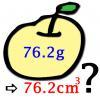More or Less?

Age 14 to 16 Challenge Level:

Are these estimates of physical quantities accurate?Quorum-sensing

Age 16 to 18 Short Challenge Level:

This problem explores the biology behind Rudolph's glowing red nose.Biology Measurement Challenge

Age 14 to 16 Challenge Level:

Analyse these beautiful biological images and attempt to rank them in size order.Over-booking

Age 16 to 18 Challenge Level:

The probability that a passenger books a flight and does not turn up is 0.05. For an aeroplane with 400 seats how many tickets can be sold so that only 1% of flights are over-booked?What Do Functions Do for Tiny X?

Age 16 to 18 Challenge Level:

Looking at small values of functions. Motivating the existence of the Taylor expansion.Counting Dolphins

Age 14 to 16 Challenge Level:

How would you go about estimating populations of dolphins?Reaction Rates

Age 16 to 18 Challenge Level:

Explore the possibilities for reaction rates versus concentrations with this non-linear differential equationThe Monte Carlo Method

Age 16 to 18 Challenge Level:

Estimate areas using random gridsDebt Race

Age 16 to 18 Challenge Level:

Who will be the first investor to pay off their debt?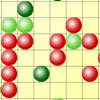Epidemic Modelling

Age 14 to 18 Challenge Level:

Use the computer to model an epidemic. Try out public health policies to control the spread of the epidemic, to minimise the number of sick days and deaths.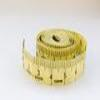Bigger or Smaller?

Age 14 to 16 Challenge Level:

When you change the units, do the numbers get bigger or smaller?Real-life Equations

Age 16 to 18 Challenge Level:

Here are several equations from real life. Can you work out which measurements are possible from each equation?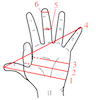Age 14 to 16 Challenge Level:

Which units would you choose best to fit these situations?Nutrition and Cycling

Age 14 to 16 Challenge Level:

Andy wants to cycle from Land's End to John o'Groats. Will he be able to eat enough to keep him going?Electric Kettle

Age 14 to 16 Challenge Level:

Explore the relationship between resistance and temperature